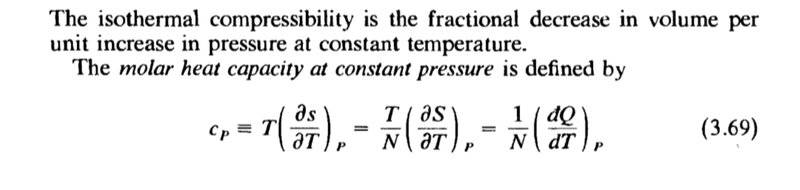# How is the derivative of an inexact differential defined?

• I
• spin_100

#### spin_100This is from Callen's thermodynamics. What does the differentiation with respect to T means for an inexact differential like dQ. Also why is T treated as a constant if we start by replacing dQ by TdS? Any references to the relevant mathematics will be much appreciated.

This is from Callen's thermodynamics. What does the differentiation with respect to T means for an inexact differential like dQ.
I am a little rusty but I think that this should not be interpreted as a differentiation but as a ratio of two incremental changes in values. In this case the change in the heat of a system d'Q * that occurs when the temperature increases by dT at a temperature of T.
$$\frac{d'Q}{dt }\neq \frac{d}{dt}\left ( Q \right )$$

* Thermodynamics by Sears always distinguishes inexact differentials with a prime superscript on d indicating a small change in the value of the quantity..

this should not be interpreted as a differentiation but as a ratio of two incremental changes in values.
Exactly.

Also why is T treated as a constant if we start by replacing dQ by TdS?
The formula ##d'Q=TdS## does not imply that ##T## is constant.

For analogy, consider classical mechanics of a particle moving in one dimension. The infinitesimal path ##dx## during the time ##dt## is ##dx=vdt##, but it does not imply that the velocity ##v(t)## is constant. Instead, it means that ##v(t)## is defined as
$$v(t)=\frac{dx(t)}{dt}$$
which physicists write in the infinitesimal form ##dx=vdt##.

Indeed, similarly to ##v##, the ##T## can also be thought of as defined by a derivative formula. But it is not ##T=d'Q/dS## or ##T=dQ/dS##, because such things are not defined as derivatives. Instead, starting from the 1st law of thermodynamics
$$dU=TdS-PdV$$
we see that ##U## must be a function of ##S## and ##V##, because then we have the mathematical identity
$$dU(S,V)=\left( \frac{\partial U(S,V)}{\partial S} \right)_V dS + \left( \frac{\partial U(S,V)}{\partial V} \right)_S dV$$
which is compatible with the 1st law above. The compatibility implies that ##T## can be defined as
$$T(S,V)=\left( \frac{\partial U(S,V)}{\partial S} \right)_V$$
which is a function of ##S## and ##V##, not a constant.

Last edited:
•gleem and PeroK